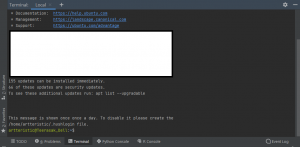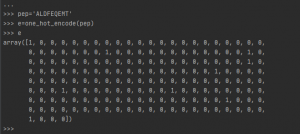# Python programming for machine learning

Asst. Prof. Teerasak E-kobon

8 February 2021

Machine learning (ML) is the approach to get the computer to program itself using the data and the output. The ML has several applications including ranking the pages on the web search, drug design, handling uncertain circumstance for the robots, and extracting values from the social network data. Three components of the MLs are (1) representation which is the way to represent the knowledge (models and algorithms), (2) evaluation which will evaluate the model or algorithm (accuracy, prediction, recall) of the candidate program, and (3) optimization which is the way to generate the program or the search process. Basically, four types of the MLs have been used in many area: (1) supervised learning which trains the model by the desired output, (2) unsupervised learning which trains the model not to include the desired output, (3) semi-supervised learning which trains the model to include a few desired outputs, and (4) reinforcement learning which has rewards for a sequence of actions.

To start ML programming, the programmer should understand the prior knowledge and set the goal explicitly. Then the data will be prepared which includes the data selection, integration, cleaning, and pre-processing before trying several learning models. Once optimized, the programmer will interpret the results and deploy the discovered knowledge.

This chapter will introduce a stepwise demonstration to use the ML concept with Python programming. The example is linked to the binding between a peptide and the MHC molecules for the antigen presentation and T-cell immune response. Many studies have been conducted on this problem and showed that the binding of the MHC class I depended on the peptide length between 8-11 amino acids. Several peptides were tested for the binding affinity with the MHC class I molecules. Bioinformatician will be able to use this knowledge and build the ML prediction model to use with the new peptides.

First of all, students will have to install the Ubuntu application on your Windows OS system because one of the Python modules (epitopepredict) will not work on the Windows OS. This Ubuntu application allows us to enumerate the Ubuntu OS on our Windows system. The pip command can be used to install Python modules on Ubuntu. If succeeded, you can call the Ubuntu application from the command prompt terminal as shown in Figure 1.Figure 1 The calling of Ubuntu application via the command prompt terminal within the PyCharm

After the Ubuntu environment is initiated, you can open Python terminal under this new environment by typing python3 in the shell command line and begin importing the required modules. On this shell terminal, you will have to input one command at a time.

Example 1 Commands to import required modules

artteristic@Teerasak_Dell:~\$ python3
Python 3.8.5 (default, Jul 28 2020, 12:59:40)
[GCC 9.3.0] on linux
>>> import os, sys, math
>>> import numpy as np
>>> import pandas as pd
>>> import matplotlib as mpl
>>> import matplotlib.pyplot as plt
>>> import seaborn as sns
>>> sns.set_context("notebook", font_scale=1.4)
>>> import epitopepredict as ep

The first process for beginning machine learning for this problem is the peptide representation of the peptide sequences into a suitable format as a matrix in this case as in the second example and visualized the matrix in Figure 2.

Example 2 The conversion of peptide sequence to a two-dimensional matrix

```codes = ['A', 'C', 'D', 'E', 'F', 'G', 'H', 'I', 'K', 'L',
'M', 'N', 'P', 'Q', 'R', 'S', 'T', 'V', 'W', 'Y']```
```def one_hot_encode(seq):
o = list(set(codes) - set(seq))
s = pd.DataFrame(list(seq))
x = pd.DataFrame(np.zeros((len(seq),len(o)),dtype=int),columns=o)
a = s.str.get_dummies(sep=',')
a = a.join(x)
a = a.sort_index(axis=1)
e = a.values.flatten()
return e```
```pep ='ALDFEQEMT'

e = one_hot_encode(pep)
```Figure 2 The peptide matrix

There are several methods of this matrix conversion. The second method uses the Fisher transformation based on the amino acid physicochemical properties.

Example 3 The second method to construct the peptide matrix

```nlf = pd.read_csv('https://raw.githubusercontent.com/dmnfarrell/epitopepredict/master/epitopepredict/mhcdata/NLF.csv',index_col=0)

def nlf_encode(seq):
x = pd.DataFrame([nlf[i] for i in seq]).reset_index(drop=True)
e = x.values.flatten()
return e

e=nlf_encode(pep)```

The third method is to use the BLOSUM matrix as in the fourth example.

Example 4 Conversion of the peptide sequence to the matrix using the BLOSUM matrix.

```blosum = ep.blosum62

def blosum_encode(seq):
#encode a peptide into blosum features
s=list(seq)
x = pd.DataFrame([blosum[i] for i in seq]).reset_index(drop=True)
e = x.values.flatten()
return e

e = blosum_encode(pep)```

Question 1 The fourth example uses the BLOSUM matrix from the ep object that instantiated from the epitopepredict module. If this module is unavailable, please write the codes to create this BLOSUM matrix and use it to replace the ep.blosum2 command.

Question 2 In order to use the Ubuntu environment, basic command-line skills are demanded. Please show at least 10 basic command lines in Ubuntu.

After the data representation step, we will select the model that can fit the created data feature matrix (the peptide matrix). In this case, the regression model is chosen (MLPregressor()) and will be trained with the peptide data (df) with known antigen-binding properties from the IEDB database.  The data is divided into the train and test data. The train data is used to teach the model to learn the known knowledge (reg.fit()) such as the peptide feature of the antigen-binding properties. The test data will be hidden from the model and used to check the model after being trained (reg.predict()). The three peptide matrixes (one_hot_encode, nlf_encode, and blosum_encode) can be tried with the created regression model and the best fit one will be selected to build the optimized prediction model (example 5). After evaluation, this prediction model will be used to predict the antigen-binding properties of other unknown peptides.

Example 5 Creation of the regression model using the nlf peptide encoder.

```# create the regression model
reg = MLPRegressor(hidden_layer_sizes=(20), alpha=0.01, max_iter=500,
activation='relu', solver='lbfgs', random_state=2)

df = ep.get_training_set(allele, length=9)
print (len(df))

# Encode with nlf_encode function
X = df.peptide.apply(lambda x: pd.Series(nlf_encode(x)), 1)
y = df.log50k

# split the data to train and test
X_train, X_test, y_train, y_test = train_test_split(X[1:50], y[1:50], test_size=0.1)

# fit data to the model
reg.fit(X_train, y_train)

# use the model to test with the test data
sc = reg.predict(X_test)

# produce a dataframe table that compares the true test data and the predicted data
x=pd.DataFrame(np.column_stack([y_test,sc]),columns=['test','predicted'])
x

```
```def auc_score(true,sc,cutoff=None):
if cutoff!=None:
true = (true<=cutoff).astype(int)
sc = (sc<=cutoff).astype(int)
fpr, tpr, thresholds = metrics.roc_curve(true, sc, pos_label=1)
r = metrics.auc(fpr, tpr)
#print (r)
return  r```
```# evaluate the prediction
auc_score(y_test, sc, cutoff=0.426)```

Question 3 The fifth example only shows the use of one peptide matrix (nlf_encode) in the regression model. Please write the codes to apply the other two peptide matrixes (blosum_encode and one_hot_encode) to the regression model. Which encoder should be the best that fits this data model? Explain.

Question 4 Please explain what is the importance of the auc_score() function.